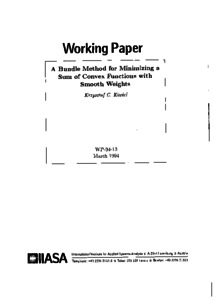# A Bundle of Method for Minimizing a Sum of Convex Functions with Smooth Weights

Kiwiel, K. (1994). A Bundle of Method for Minimizing a Sum of Convex Functions with Smooth Weights. IIASA Working Paper. IIASA, Laxenburg, Austria: WP-94-013Preview Text WP-94-013.pdf Download (435kB) | Preview

## Abstract

We give a bundle method for minimizing a (possibly nondifferentiable and nonconvex) function h(z) = sum_{i=1}^m p_i(x) f_i(x) over a closed convex set in R^n, where p_i are nonnegative and smooth and f_i are finite-valued convex. Such functions arise in certain stochastic programming problems and scenario analysis. The method finds search directions via quadratic programming, using a polyhedral model of h that involves current linearizations of p_i and polyhedral models of f_i based on their accumulated subgradients. We show that the method is globally convergent to stationary points of h. The method exploits the structure of h and hence seems more promising than general-purpose bundle methods for nonconvex minimization.

Item Type: Monograph (IIASA Working Paper) Optimization under Uncertainty (OPT) IIASA Import 15 Jan 2016 02:04 27 Aug 2021 17:14 https://pure.iiasa.ac.at/4196View Item